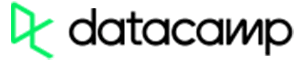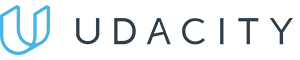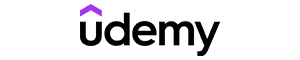Code has been added to clipboard!

# Math

Published Aug 9, 2017
Updated Oct 2, 2019

## JavaScript Math: Main Tips

• The `Math` object in JavaScript is for performing mathematical calculations.
• This object has properties and methods for getting mathematical constants. It also has operations that are time-consuming to manually write in expressions.

## Use of the Math Object: Easy Functions to Learn

The JavaScript `Math` object should not be mistaken for a constructor. It accepts `Number` type but won't work with `BigInt`.

For instance, `Math.min` JavaScript will present you with the lowest value:

Example
``Math.min(65, 951, 84, 20, -98, -235);``

Another method is the `Math.floor` in JavaScript, delivering a value rounded downwards to the closest integer.

Example
``````Math.floor(5.8);
Math.floor(3.2);``````

JavaScript also offers another function called `Math.round()` for rounding values upwards to the closest integer:

Example
``````Math.round(5.8);
Math.round(3.2);``````

You can get the square root of a specific number without having to open a separate calculator. To get JavaScript square root, you should apply the `Math.sqrt()` function.

Here is a short example, illustrating how you can make JavaScript count square root of 100:

Example
``Math.sqrt(100);``Pros
• Easy to use with a learn-by-doing approach
• Offers quality content
• Gamified in-browser coding experience
• The price matches the quality
• Suitable for learners ranging from beginner to advanced
Main Features
• Free certificates of completion
• Focused on data science skills
• Flexible learning timetablePros
• Simplistic design (no unnecessary information)
• High-quality courses (even the free ones)
• Variety of features
Main Features
• Nanodegree programs
• Suitable for enterprises
• Paid Certificates of completionPros
• Easy to navigate
• No technical issues
• Seems to care about its users
Main Features
• Huge variety of courses
• 30-day refund policy
• Free certificates of completion

## List of Object Properties

One important thing to note is that the `Math` object does not have a `constructor`. However, it has static properties.

Property Description
E Return Euler's number (approximately 2.718).
LN2 Return natural logarithm of 2 (approximately 0.693).
LN10 Return natural logarithm of 10 (approximately 2.302).
LOG2E Return base-2 logarithm of E (approximately 1.442).
LOG10E Return base-10 logarithm of E (approximately 0.434).
PI Return PI (approximately 3.1416).
SQRT1_2 Return square root of 1/2 (approximately 0.707).
SQRT2 Return square root of 2 (approximately 1.414).

Tip: you can call these properties and functions below by applying Math as the object instead of creating it.

## Functions to Apply

Here is a cheat-sheet, containing all methods for more successful and quick calculations.

Note: results of most of these functions depend on browsers, operating systems and architectures used.

Method Description
abs(x) Return absolute value of x.
acos(x) Return arccosine of x in radians.
asin(x) Return arcsine of x in radians.
atan(x) Return arctangent of x as numeric value between -PI/2 and PI/2 radians.
atan2(y,x) Return arctangent of quotient of its arguments.
ceil(x) Return x rounded upwards to the nearest integer.
cos(x) Return cosine of x (x is in radians).
exp(x) Return value of Ex.
floor(x) Return x rounded downwards to the nearest integer.
log(x) Return natural logarithm (base E) of x.
max(x,y,z,...,n) Return number with the highest value.
min(x,y,z,...,n) Return number with the lowest value.
pow(x,y) Return value of x to power of y.
random() Return a random number between 0 to 1.
round(x) Round x to the nearest integer.
sin(x) Return sine of x (x is in radians).
sqrt(x) Return square root of x.
tan(x) Return tangent of angle.

Remember: sin(), cos(), tan(), asin(), acos(), atan(), atan2() functions return or should be used with angles in radians. For conversion from radians to degrees, you should divide by Math.PI / 180. To perform the opposite, multiply instead of dividing.

Days
Hours
Minutes
Seconds